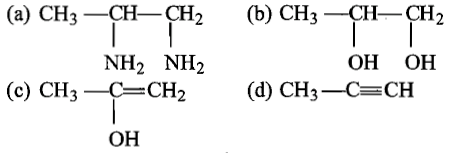Which of the following are known as mercaptans?

1. Thio-alcohols

2. Thio-ethers

3. Thio-aldehydes

4. Thio-acids

Concept Questions :-

Ethers: Preparation, Physical & Chemical Properties, Uses
High Yielding Test Series + Question Bank - NEET 2020

Difficulty Level:

Glycol condenses with ketones to give:

1. cyclic acetals                   2. cyclic ketals

3. acetaldehyde                   4. oxalic acid

Concept Questions :-

Alcohols: Preparation, Physical and Chemical Properties
High Yielding Test Series + Question Bank - NEET 2020

Difficulty Level:

Identity Z in the sequence of reactions,

CH3CH2CH=CH2$\stackrel{{\mathrm{HBr}}_{2}/{\mathrm{H}}_{2}{\mathrm{O}}_{2}}{\to }$$\stackrel{{\mathrm{C}}_{2}{\mathrm{H}}_{5}\mathrm{ONa}}{\to }$Z

(a) CH3-(CH2)3-OCH2CH3

(b) (CH3)2CH2-OCH2CH3

(c) CH3(CH2)4-OCH3

(d) CH3CH2-CH(CH3)-OCH2CH3

Concept Questions :-

Ethers: Preparation, Physical & Chemical Properties, Uses
High Yielding Test Series + Question Bank - NEET 2020

Difficulty Level:

Salol is used as:

(a) antiseptic                                (b) antipyretic

(c) both (a) and (b)                      (d) none of these

Concept Questions :-

Ethers: Preparation, Physical & Chemical Properties, Uses
High Yielding Test Series + Question Bank - NEET 2020

Difficulty Level:

Identify Z in the following series,

CH3-CH2-CH2OHX$\stackrel{{\mathrm{Br}}_{2}}{\to }$YZConcept Questions :-

Alcohols: Preparation, Physical and Chemical Properties
High Yielding Test Series + Question Bank - NEET 2020

Difficulty Level:

Ethyl propanoate on reduction with LiAlH4 yields:

1. methanol

2. ethanol and propanol

3. propane

4. mixture of ethanol and methanol

Concept Questions :-

Ethers: Preparation, Physical & Chemical Properties, Uses
High Yielding Test Series + Question Bank - NEET 2020

Difficulty Level:

Phenol does not react with:

(a) Na2CO                                (b) NaOH

(c) NaHCO3                                 (d) KOH

Concept Questions :-

Phenols: Preparation, Properties, Acidic Nature of Phenol
High Yielding Test Series + Question Bank - NEET 2020

Difficulty Level:

Which alcohol is most acidic?

1. Methanol

2. Ethanol

3. Isopropyl alcohol

4. t-butyl alcohol

Concept Questions :-

Alcohols: Preparation, Physical and Chemical Properties
High Yielding Test Series + Question Bank - NEET 2020

Difficulty Level:

Consider the following reaction,

Phenol$\stackrel{\mathrm{Zn}-\mathrm{dust}}{\to }$X$\underset{\mathrm{Anhy}.{\mathrm{AlCl}}_{3}}{\overset{{\mathrm{CH}}_{3}\mathrm{Cl}}{\to }}$Y$\stackrel{\mathrm{Alk}.{\mathrm{KMnO}}_{4}}{\to }$Z

The product Z is

(a) toluene

(b) benzaldehyde

(c) benzoic acid

(d) benzene

Concept Questions :-

Phenols: Preparation, Properties, Acidic Nature of Phenol
High Yielding Test Series + Question Bank - NEET 2020

Difficulty Level:

The product in the reaction is:

C2H5OH $\stackrel{\mathrm{P}+{\mathrm{I}}_{2}}{\to }$A$\underset{\mathrm{Ether}}{\overset{\mathrm{Mg}}{\to }}$B$\stackrel{\mathrm{HCHO}}{\to }$C$\stackrel{{\mathrm{H}}_{2}\mathrm{O}}{\to }$D

1. propanal

2. butanal

3. n-butanol

4.  n-propanol

Concept Questions :-

Alcohols: Preparation, Physical and Chemical Properties